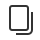• 介绍
• 设计
• 开发
• 数据
• 运营
• 第三方平台
• 社区# command.or``````    swan.cloud.init({
env: 'envId'
});
const db = swan.cloud.database();
db.collection('users')
.where({
age: _.lt(25).or(_.gt(50))
})
````````````    swan.cloud.init({
env: 'envId'
});
const db = swan.cloud.database();
db.collection('users')
.where({
age: _.or(_.lt(25), _.gt(50)) // or指令同时支持传入数组，_.or([_.lt(25), _.gt(50)])
})
````````````    swan.cloud.init({
env: 'envId'
});
const db = swan.cloud.database();
db.collection('users')
.where(_.or([
age: _.gt(25),